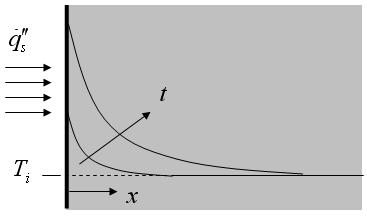Contents - Index

Uniform Heat FluxThe function semiinf2(T_i,q``_dot_s,k,alpha,x,time) returns the temperature for a given position and time within a semi-infinite body exposed to a uniform surface heat flux using the relation provided in Table 3-2 of Nellis and Klein.

Inputs:
T_i - initial temperature of the solid  [C] or [K]
q``_dot_s - surface heat flux [W/m^2]
k - thermal conductivity [W/m-K]
alpha - thermal diffusivity [m^2/s]
x - perpendicular distance from surface [m]
time - time relative to flux application (flux begins at t=0) [s]

This function can be used with English units set in EES.  In this case, T_i is in [F] or [R],  q``_dot_s  is in Btu/hr-ft^2-R, k is in Btu/hr-ft, alpha is in ft^2/hr and x is in [ft].  Time must be in s.

Example:
\$UnitSystem SI K Pa J
\$VarInfo T units=K
T_i=378[K]
q``_dot_s=2500 [W/m^2]
alpha=0.00010 [m^2/s]
k=50 [W/m-K]
x=0.001 [m]
time=200 [s]
T=semiinf2(T_i,q``_dot_s,k,alpha,x,time)

{Solution:
T=385.9 [K]}

Transient Conduction Index